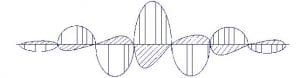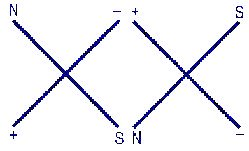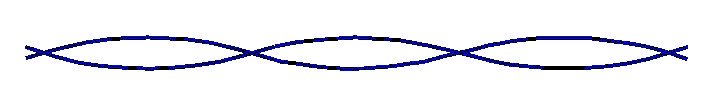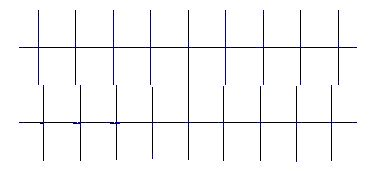01270 747 008 (UK)

# A Possible Sub-Structure for the Neutrino

By Richard D. Fuerle

This article was submitted as a term paper for a course in Particle Physics, Physics 521, given in the Spring semester by Professor Doreen O. Wackeroth, whose guidance and assistance is gratefully acknowledged. However, the paper was not accepted as I could not explain how two photons could bind together or how angular momentum would be preserved if two photons combined to form a neutrino. Possible explanations have since been added to this article.

Abstract

A possible sub-structure for the electron neutrino is suggested. The neutrino may consist of two aligned photons with their magnetic and electric fields interlocked. That structure is consistent with the properties of the neutrino, including its tiny mass, velocity slightly less than the speed of light, helicity, internal frequency, failure to decay, stability, and unreactiveness. Of particular interest is the recently discovered phenomenon of neutrino oscillation, which requires a single neutrino to have two slightly different wavelengths, also consistent with the proposed structure. Calculations based on this structure yield reasonable results. A few experimentally testable predictions are made.

Introduction
As science has progressed through the ages, matter has been shown to be made of molecules, molecules of atoms, atoms of electrons and nucleons, and nucleons of quarks. Including anti-particles, there are still at least 61 “elementary” particles.1 A number of current theories, such as string theory and M-brane theory, propose sub-structures for so-called elementary particles.2 Models of neutrinos that have mass have been constructed, but none are theoretically compelling.3 The neutrino, long considered to be an elementary particle, has a number of unique properties that are suggestive of an underlying structure. It is proposed that these properties are consistent with an sub-structure consisting of two aligned interlocked photons with axes slightly twisted. The discussion will be limited to the electron neutrino, which will hereinafter be referred to simply as “the neutrino.”

Properties
Neutrinos have some very unusual and unique properties that have not been explained.

Classification
Since the neutrino has a half integer spin of ½, it is a fermion, along with other matter particles, such as the quarks, mesons, and hadrons. It is a member of the family of leptons, which includes the electron, the muon, the tau, the electron neutrino, the muon neutrino, and the tau neutrino, each of which has an anti-particle.

Forces
The neutrino feels only the weak force and gravity.

Flavors
Neutrinos come in three “flavors,” the electron neutrino, ve, the muon neutrino, vµ, and the tau neutrino, vt Each flavor is associated with the corresponding charged electron. That is, whenever an electron is created with a neutrino, it is always an electron neutrino and not a muon neutrino or a tau neutrino, and whenever an electron neutrino collides with matter, it always creates an electron, never a muon or a tau, and similarly for the other flavors.

Mass
Because no right-handed neutrinos and no left-handed anti-neutrinos have been found, it was concluded that neutrinos have no rest mass. The reason is that if they have a rest mass, special relativity requires that they must have a velocity less than c, the speed of light. In principle, then, a observer going faster than the neutrino would see a right-handed neutrino moving away from him, and no right-handed neutrinos are known to exist.4 Neutrino oscillation, however, meant that neutrinos have mass,5 as will be hereinafter explained. The electron neutrino has a rest mass of <5.1 eV/c2; the muon and tau neutrinos are more massive.

Velocity
While neutrinos were at first thought to travel at the speed of light, c, it is now known that their speed is very slightly less than c. In 1987, a supernova exploded 180,000 light-years away. Almost 30 minutes after telescopes detected light from the blast, neutrino detectors detected a torrent of neutrinos. The time lag gives a neutrino from that explosion a velocity of 0,9999999997 c (Footnote 6). Unlike other fermions, such as the electron, the neutrino does not have to be accelerated with huge amounts of energy to reach this extreme velocity – it travels at just under c ab initio, without any acceleration required. Neutrinos are believed to have a continuum of velocities7 that may depend upon their energy.8 Like the photon, the neutrino is never found at rest, or even moving at a velocity much less than c.

Neutrality
Unlike other elementary particles, which are either charged or are composite particles made up of equal amounts of positive and negative charges (e.g., the neutron), the neutrino is completely neutral. Not only is it neutral, but it is the most neutral particle known, more neutral than even the photon, as the photon has an electromagnetic field which the neutrino lacks.

Magnetic Moment
Counterintuitively for a neutral particle, the neutrino has a tiny magnetic moment of about 10-11. This is believed to be due to a loop effect, where the neutrino generates a virtual W particle, which annihilates back into the neutrino.

Anti-neutrino and Helicity
Unlike all other fermions, where the anti-particle differs from the particle only in charge, the anti-neutrino does not differ in charge (neutrinos are neutral) but in helicity. All neutrinos are left handed and all anti-neutrinos are right handed.

Decay and Stability
Neutrinos have since their conception been considered to be elementary particles.9 Since they have the smallest rest mass of any known fermion, there is no other fermion that they can decay into, and they are not known to decay.

Of all the particles, none is more stable than the neutrino. It is capable of passing through 130 light years of solid iron before interacting with another particle.10 And, unlike all the other particles, including even the electron, it is so stable that it will not annihilate even with its own antiparticle.

Energy
While the energy of a fermion depends upon its velocity, the energy of a neutrino is not due (entirely) to its kinetic energy, but also to an internal frequency, v, according to the equation E = hv. Neutrinos, like photons, have a continuum of energies proportional to their frequency.11

Proposed Sub-Structure
A photon has an electromagnetic field consisting of electric and magnetic fields at right angles. The fluctuating fields are a fixed distance apart, equal to the wavelength:While the exact structure of the photon is not known, it is generally pictured as consisting of several complete cycles with the amplitudes of the cycles diminishing at the ends of the wave train.12

The properties of the neutrino may possibly be explained if the neutrino consisted of two intertwined photons moving together with their electric and magnetic fields interlocked. (A good analogy is double stranded DNA, where the sense and antisense strands bind to each other through base pairs.) An end view of the two photons would show the attraction between opposing electric and magnetic fields:As can be seen in the above drawing, in order for the electric fields to line up positive- to-negative and the magnetic fields to line up north-to-south, it is necessary for one of the photons to be rotated 90° and for the relationship between the electric and magnetic fields to be reversed.

While photons do not bind directly to other photons as there is no carrier of a binding force, photons can fluctuate into a hadronic state via the reaction by forming a quark-antiquark pair, and they can bind the two photons together.13 (A fluctuation into an electron-positron pair may also produce binding.) A fluctuation to a quark-antiquark pair or to an electron-positron pair would also account for the slight magnetic moment of the neutrino.

In addition to the problem of how two photons would bind together, the reaction of two photons with a spin of 1 to form a neutrino with a spin of ½ does not conserve angular momentum. While angular momentum is certainly conserved, it is possible that the neutrino is not formed by that reaction, but by a reaction involving another particle that carries off angular momentum.

The interlocking of these fields should not only cancel any external or net electric and magnetic fields that the two photons would otherwise display, but should bind the two photons together through the powerful electromagnetic force, giving the neutrino its extraordinary stability.14 If the average distance between the centers of the fields is small, the electromagnetic force would be very large, ample to prevent the neutrino from breaking apart in even the most energetic collisions.

It is further proposed that the axes of the two photons are not linear, but spiral slightly around each other (as a DNA double helix does) as shown in the following drawing of the two axesThe slight rotation of the axes may be due to the reduced amplitude of the photons at the ends of their wave trains, which may pull the trains together at their ends, twisting the axes of the photons. Also, if the two photons do not have exactly the same wavelengths, the fields may be directly opposite at the centers of the wave trains, but offset slightly at the ends, which may twist their axes.
There will be, of course, two possible configurations for the neutrino, a clockwise twist and an counterclockwise twist, corresponding to an anti-neutrino and a neutrino, and this would explain why the anti-neutrino differs from the neutrino in helicity rather than in charge. There is no reason to expect that the conflicting helicities of the neutrino and the antineutrino would result in the disengagement of the photon pairs that comprise them, should the neutrino collide with its anti-neutrino, and no such annihilation has been observed. This would be consistent with the proposal that the neutrino is a Majorana particle and does not have an anti-particle.

If the axes are twisted slightly, then other properties of the neutrino may be explained. First, while each photon would continue to move at the speed of light, each would now follow a helical path instead of a linear path. Thus, the photon velocity c will have a forward component and a rotational component. The forward component of the velocity of the photon will be equal to the velocity of the neutrino, which will be slightly less than c.

If the proposed structure is correct, the number of helical rotations per linear distance of neutrino travel should be proportional to the frequency of the photons because the amount of twisting should depend upon the binding force between the two photons, which should increase with frequency. That is, if the two photons that comprise a neutrino have a long wavelength, they should travel a long linear distance for each helical rotation and if they have a short wavelength (high frequency) they should have many rotations in that same distance.

Moreover, the rotational component of the velocities of the photons in the intertwined pair will be observed as rest mass.15This can be most easily seen by imagining for a moment that the entire velocity of the photons is rotational. In that case, the neutrino would be at rest, relative to the laboratory frame of reference, and its entire energy could be measured by weighing it. In other words, its entire energy would be in the form of its rest mass.16 If that is true, then when the rotational component of the velocity of the photons in the neutrino is tiny, the rest mass would be correspondingly tiny.

In order to match the electromagnetic fields of the two photons, the two photons will have to have approximately the same wavelengths. Since the light spectrum is continuous, this means that neutrinos can also have a continuous spectrum of wavelengths, which explains the internal frequency of the neutrino that gives neutrinos different energies.

The phenomenon of neutrino oscillation strongly supports the proposed sub- structure. Neutrino oscillation requires the neutrino to consist of two waves that do not have exactly the same wavelength.17 Two photons of slightly different wavelengths would meet that requirement. The following side view shows the axes of two photons that differ slightly in wavelength:The two slightly-differing wavelengths of the photons in the neutrino interfere slightly, which creates an oscillation between two different frequencies,18 which would correspond to the different flavors of neutrino. While the electromagnetic coupling between the two photons that comprise a neutrino would be maximized if the two photons had exactly the same wavelength, a small difference in wavelength would not reduce the binding force much, especially if the wave train is not very long.

Predictions
This explanation for the sub-structure of the neutrino leads to several predictions that it may be possible to test experimentally. Both the velocity and the rest mass of the neutrino should be directly proportional to the number of rotations (helical turns) per unit of linear distance traveled. That is, if there is no rotation, the linear velocity of the neutrino should be c and its rest mass should be zero and, if the entire velocity component of the two photons is rotational, the linear velocity should be zero and the rest mass should equal the entire energy of the neutrino. Therefore, if the number of rotations per unit of linear distance traveled is proportional to the frequency of the neutrino, the linear velocity and the rest mass should also be proportional to the frequency.18 This would make the neutrino unique in yet another way, being the only particle having a continuum of rest masses and a continuum of velocities19 proportional to that rest mass.

Also, since both of the photons in a neutrino must have wavelengths that are nearly the same in order to bind together, this suggests that if a laser beam is split, one portion is rotated 90° and the two portions are recombined colinearly with reversed electric-magnetic field positions (e.g., possibly by moving in opposite directions), it may be possible to create neutrinos from photons.20 This would not violate conservation of angular momentum if (1) the photons move in opposite directions, (2) a photon moving in the opposite direction had an angular momentum of -1, and (3) a neutrino- antineutrino pair was created.

Since neutrino oscillations require that the two photons have slightly different wavelengths, it may be possible to produce neutrinos that do not oscillate by using photons that have exactly the same wavelengths. And, unless there is a quantum effect,21 unique neutrinos other than the electron, muon, and tau neutrinos might be created by controlling the difference between the wavelengths.

Calculations
In accordance with the proposed neutrino sub-structure, the ratio of the rest mass of the neutrino to its energy should be the same as the ratio (c – v)/c, where “v” is the linear velocity of the neutrino. As a particular example to test the reasonableness of the proposed sub-structure, let us use a neutrino linear velocity of 0.9999999997 c22 and an energy of 1 MeV23. This gives a rest mass of about 3×10-4 eV/c2 (3×10-10/ 1×106), which is within the known neutrino rest mass range of <5.1 eV/c2. Using c = 3×108 m/s, that same linear velocity suggests that each photon in a neutrino will travel a linear distance of 2.9999999991×108 meters in a second (0.9999999997 x (3×108)) and a rotational distance of 0.09 meters in that same second (3×108 – 2.9999999991×108). A rest mass of 3×10-4 eV/c2 suggests a frequency of 7.2×1010 cycles/s (E/h = (3×10-4 eV/c2) / (4.14×10-15eV•s)), which gives a wavelength (c/v) of 4.1×10-3 meters. Dividing by 2pi gives a radius of 6.6×10-4 meters, suggesting the distance between each photon and the neutrino axis. The wavelength is the circumference of the circle, so there would be 137 rotations per second (0.09/6.6×10-4). In this example, that would mean that the two photons rotated once for every 2,200,000 meters (3×108/137) or 1400 miles that the neutrino traveled.

1. Veltman, Martinus, Facts and Mysteries in Elementary Particle Physics, World Scientific Publishing Co Pte. Ltd. (2003), page 69.
2. Eichten, E. et al., “New Tests for Quark and Lepton Substructure,” Fermilab- Pub-83-015-T; Peskin, Michael E., “The Compositeness of Quarks and Leptons,” Proceedings of the 1981 International Symposium on Lepton and Photon Interactions at High Energy (1981).
3. Kane, Gordon, Modern Elementary? Particle Physics, Westview Press (1993).
4. Muirayama, Hitoshi, “The Origin of Neutrino Mass,” Physics World, May, 2002.
5. Kane, Gordon, ibid, pages 303-311. Seiden, Abraham, Particle Physics, Addison Wesley (2005), pages 333-345. “Neutrino Oscillations,” http://ww.ps.uci.edu/-superk/oscillation.html; Murayama, Hitoshi, “Neutrino Physics: Evidence for Neutrino Mass,” http://hitoshi.berkeley.edu/neutrino/neutrino3.html (2002).
6. The Five Common Particles, Summary of Elementary Particle Physics, Northwestern University, http://www.physics, northwestern.edu/classes/2004Spring/Phyx103/fiveparticles.doc
7. Okun et al., “Comment on Equal Velocity Assumption for Neutrino Oscillations,” Modern Physics Letters A, argue that “equal velocity is forbidden by simple kinematic considerations.”
8. G.R. Kalbfleisch, The Velocity of the Neutrino, Brookhaven National Laboratory (May, 1975) speculates that there may be “a dependence of the velocity upon the energy of the neutrino.”
9. Veltman, Martinus, ibid, page 69. 10. ?Concepts of Modern Physics, Arthur Beiser (1967). 11. ?Reynolds, David J., “A Solution for the Solar Neutrino Problem,” http://www.btinternet.com/~david.reynolds1/ “Wave particle duality dictates that a neutrino will, in its own frame of reference, have a frequency related to its rest mass via Plancks constant.”
12. In addition to the conventional structure shown in the drawing, where the electric cycles alternate positive and negative and the magnetic cycles alternate north and south, it is possible that the positive and negative electric cycles (and the north and south magnetic cycles) may be in opposition, and that structure may even be more likely.
13. Particle Physics Booklet, Particle Data Group, Lawrence Berkeley National Laboratory, July, 2004.
14. The fields of ordinary photons do not interlock because the fields are waves that superimpose. However, because the neutrino velocity is less than c, the fields have fermion properties and can act on other fields, just as the fields of electrons.
15. Sternglass, Ernest J., Before the Big Bang, Four Walls Eight Windows (1997), pages 135, 158.
16. This reasoning suggests the source of mass for all particles.
17. Casper, Dave, “Neutrino Oscillations,” http://www.ps.uci.edu/~superk/oscillation.html
18. Reynolds, David J., “A Solution for the Solar Neutrino Problem,” http://www.btinternet.com/~david.reynolds1/ “Wave particle duality dictates that a neutrino will, in its own frame of reference, have a frequency related to its rest mass via Plancks constant.”
19. Okun et al., “Comment on Equal Velocity Assumption for Neutrino Oscillations,” Modern Physics Letters A, argue that “equal velocity is forbidden by simple kinematic considerations.”
G.R. Kalbfleisch, The Velocity of the Neutrino, Brookhaven National Laboratory (May, 1975) speculates that there may be “a dependence of the velocity upon the energy of the neutrino.”
20. Light by light scattering has been observed as a quantum mechanical effect. Veltman, Martinus, ibid., page 252.
21. Quantum effects may permit only certain frequencies. In addition, the twist in the axes of the photons will introduce a stress and at high frequencies the stress may be too great for the neutrino to form.
22. “The Five Common Particles, Summary of Elementary Particle Physics,” Northwestern University, http://www.physics, northwestern.edu/classes/2004Spring/Phyx103/fiveparticles.doc
23. Since the mass of an electron is 0.511 MeV, one might suspect that the energy of each photon in the neutrino would also be about 0.511 MeV, especially since an electron and a positron annihilate to form two photons, each with an energy of 0.511

Next Page: Photon Violation Spectroscopy
Previous Page: A Theory Of Everything

## One Comment

1.I noted in the beginning of my article that it was not accepted for a course in Particle Physics because I could not explain how two photons could bind together. An “optical force” has now been discovered that is perpendicular to the direction of a light bean, is stronger at short distances, and can be attractive or repulsive. This “optical force” should provide the needed explanation. An article on it can be read here: Tunable bipolar optical interactions between guided lightwaves,
Mo Li, W. H. P. Pernice & H. X. Tang Nature Photonics 3, 464 – 468 (2009) Published online: 13 July 2009 | doi:10.1038/nphoton.2009.116

http://www.nature.com/nphoton/journal/v3/n8/abs/nphoton.2009.116.html